Next: Gaussian Intrinsic Resolution and Up: 4.1 Spatial Resolution Previous: 4.1 Spatial Resolution

## Focus and Penetration Effects

The design distance between the XMA and PSPC (the window of which is bowed slightly out due the internal pressure of the counter gas) is such that photons of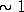keV on-axis source are brought to a focus (along a surface of a cone of half-angle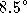) at an optimum depth within the PSPC drift region such that the Gaussian spatial distribution of pulses on the cathode wires is minimized. Consequently the image of a point source is sharpest at 1keV.

The analytical form derived for the fraction of photons within this component as a function of energy is given by whichever is the smaller of the following two terms: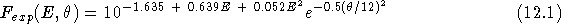or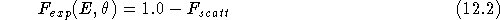Equation 4.2 is necessary to avoid the ``exponential artifact''of [Hasinger et al.1992] which made the PSF parameterization invalid above 2 keV.

The combined contribution of focussing and the finite penetration of photons into the counter on the PSF can be modelled as an exponential function where r is the radial distance from the centroid position of the radial profile, in arc minutes.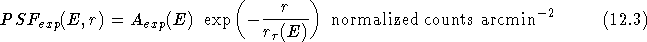The e-folding angle,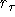, was found to be the same as for the on-axis case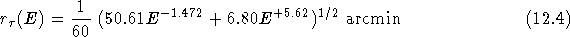The normalization,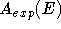, is given by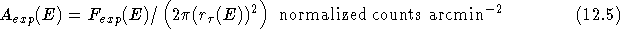and as for the other components, has been normalized such that the integral of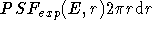over the range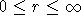equals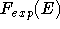.

If you have problems/suggestions please send mail to rosat_svc@mpe-garching.mpg.de